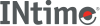﻿ lldivINtime SDK Help
lldiv

Divides 64-bit numerator by denominator, and computes the quotient and remainder.

```#include <stdlib.h>

lldiv_t lldiv (long64 numer, long64 denom);
```

#### Parameters

`numer`
Numerator.
`denom`
Denominator. If 0, errno is set to EDOM and 0 returned for quotient and remainder.

#### Remarks

The sign of the quotient is the same as that of the mathematical quotient. Its absolute value is the largest long integer that is less than the absolute value of the mathematical quotient.

lldiv is similar to ldiv and div, except that the arguments and the members of the returned structure are 64-bit long integers.

#### Return Values

An lldiv_t structure, comprising both the quotient and the remainder, defined in `stdlib.h`.

#### Requirements

Versions Defined in Include Link to
INtime 5.0 intime/rt/include/stdlib.h stdlib.h clib.lib